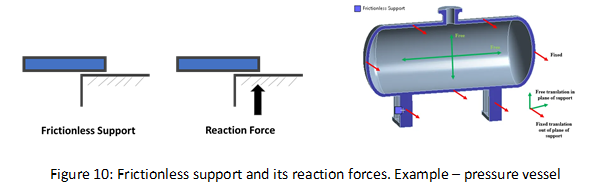# Bernoulli’s Equation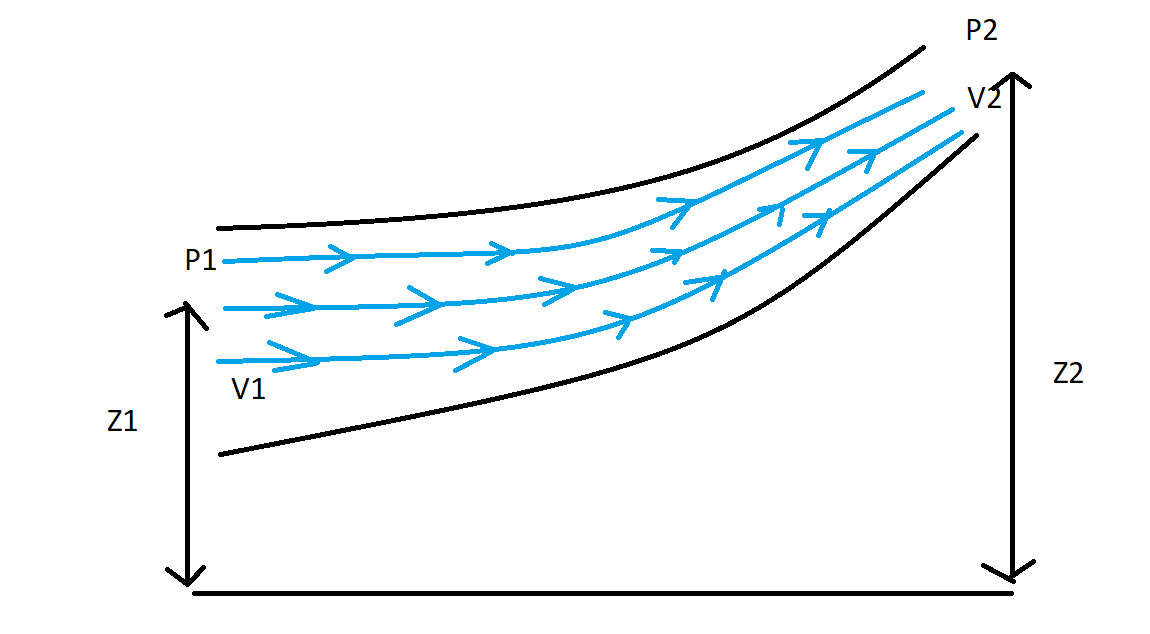## Bernoulli's Equation

Bernoulli’s Equation

One of the most interesting and important equations of fluid mechanics. It comes under the domain of fluid dynamics to be specific. Bernoulli’s equation is also sometimes treated as the conservation of energy principle. Its origin can be said as the integrated version of Euler’s equation.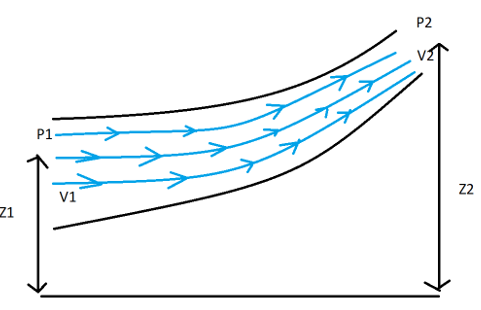Taking about the dynamics of the fluid first let us talk about the forces that are acted on the fluid in general. Following are the forces that act but vary from case to case and are also dependent on the assumptions we consider during any derivation as well as our understanding.

1. Gravity Force (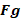)
2. Pressure Force (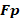)
3. Viscous Force (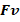)
4. Force due to compressibility (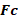)
5. Force due to turbulence (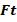)
6. Surface Tension Force (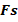)

In this case, when we consider this force then we can call it Newton’s equation of Motion.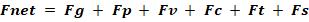In the case when all the forces except the surface tension force and compressibility forces are acted the resultant equation is termed as Reynold’s Equation.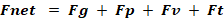Now if we talk about the case where, only gravity force, pressure force, and viscous are considered then the equation is termed as Navier Strokes Equation.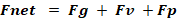Now coming back to the discussion of Euler, the forces considered during its derivations are gravity force and Pressure Force. For more details derivation of the above equations can be looked upon.

One of the most important things that need to be coupled with Bernoulli’s equation is the Continuity Equation. This conveys the message of mass conservation. In simple words, we can say that it balances the mass and keeps a check on it so that our work never fails. It means the amount of mass entering into a section and the amount of mass exiting a section should be balanced. Continuity equation helps a lot while problem-solving using Bernoulli’s equation.

ρAV = C

Where   ρ = Density of the fluid

A  = Area of the Cross- section

V = Velocity of the Fluid

After a brief overview of the different kinds of forces that are considered during different equations, lets look in the assumptions of Bernoulli’s Equation. We will, for now, keep our discussion limited to Incompressible Fluids otherwise the level of the complexities will increase

The assumptions are :

1. Flow is always considered on a streamline
2. The Fluid is assumed to be steady
3. Effect of irreversibilities, viscous forces, and friction are not considered here
4. The fluid is always treated as incompressible ( density = constant )

The derivation of Bernoulli’s equation revolves around the linear momentum principle.

Bernoulli’s equation is basically the combination of pressure, velocity and elevation. Each term of the equation is generally referred to as head namely, Pressure head, Kinetic Energy and Elevation head. These three terms are added and hence net conservation of energy takes place. This equation finds its application in many fields including Aerodynamics.

P + ρgh  +  (½)ρv2 = C

Where                        P = Static Pressure

ρgh = Potential Energy

(½)ρv2  = Kinetic Energy

Lets now look into each term of Bernoulli’s equation individually

Pressure Head: Pressure Energy per unit weight, P here means the static pressure. Energy changes and the head changes because of the actual pressure.

Velocity Head: Kinetic Energy per unit mass, The changes in the head take place because of the flow of the fluid.

Elevation Head: Potential Energy per unit mass, the change in the head takes place because of the elevation of the 2 points which are taken into consideration.

Every equation comes with a set of warning labels in the form of assumptions, as it puts a limit on its application and usage. These also help us to not land on wrong results. However there are very idealistic assumptions taken in the derivation of Bernoulli’s equation, we tend to find situations where we can easily apply these results.

As the frictions remain unavoidable in most cases, Bernoulli’s equation is also adjusted a little to help us in those situations.

Let us now see some of the examples where we should not apply this equation.

Case 1: We should never apply them in the very long narrow flow passage, the reason is because of the more length and less effective diameter the friction becomes really significant.

Case 2: Near the boundary layer, also the friction becomes very high and the generation of shear stress and rotational fluid happens

Case 3: In the case of diverging sections care has to be taken as in the diverging section the chances of flow separation are very high which can give rise to wake formation. The generated wake gives rise to irreversibility and high viscosity.

Case 4: Whenever Mach number is greater than 0.3, below 0.3 the fluid is assumed to be incompressible. Above 0.3, this assumption starts to fail as fluid tends to move towards compressibility (i.e. there is a significant change in the density)

Case 5: The sections where temperature changes are significant. The temperature change causes an effect on the density, which makes fluid compressible.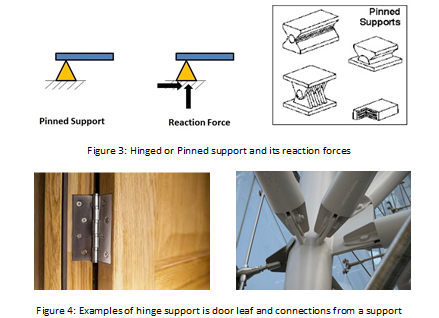1. Roller Support:

It is a support that is free to rotate and translate along the surface on which they rest. The surface on which the roller supports are installed may be horizontal, vertical, and inclined to any angle. They resist only vertical loads.  The roller supports has only one reaction, this reaction acts perpendicular to the surface and away from it. The reaction offered by the roller support is shown in figure 5. In other words, the roller supports are unable to resist the lateral loads (the lateral loads are the live loads whose main components are horizontal forces). The best example of roller support is the roller skates as in fig 6 along with some other common applications. The roller skates resist the vertical loads of the persons standing on them. When the lateral loads are applied by the persons, then it starts translating. The translation is due to its inability to resist the lateral loads.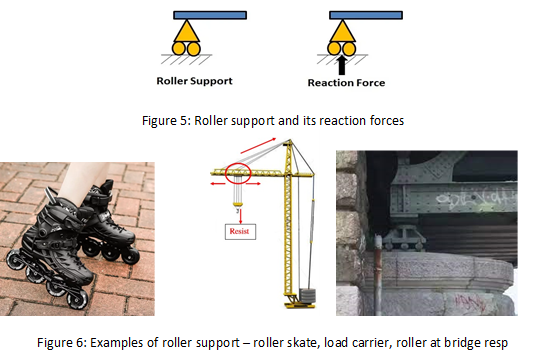1. Rocker support:

Rocker support is similar to roller support. It also resists vertical force and allows horizontal translation and rotation as in fig 7. But in this case, horizontal movement is due to the curved surface provided at the bottom as shown below figure. So, the amount of horizontal movement is limited in this case as shown in fig 7.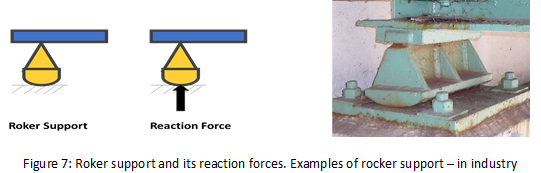A link has two hinges, one at each end. The link is supported and allows rotation and translation perpendicular to the direction of the link only. It does not allow translation in the direction of the link. It has a single linear resultant force component in the direction of the link which can be resolved into vertical and horizontal components. In other words, the reaction force of a link is in the direction of the link, along its longitudinal axis. The support and the reactions to the link constraint are shown in figure 8. The best example of link support is pliers and in most locomotives, it is used to connect the parts as shown in figure 8.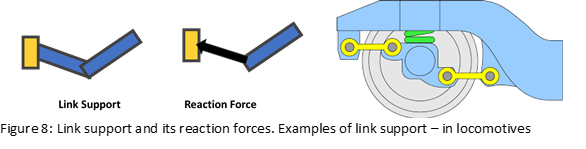1. Simple supports

Simple support is just support on which a structural member rests on an external structure. They cannot resist lateral movement and moment like roller supports. They only resist vertical movement of support with the help of gravity. The horizontal or lateral movement allowed is up to a limited extent and after that, the structure loses its support. It’s just like a brick resting longitudinally on two bricks. Another example is a plank of wood resting on two concrete blocks. Simple supports aren’t widely used in real-life structures. The simple support and the reactions that we get in the type of support are shown in the figure.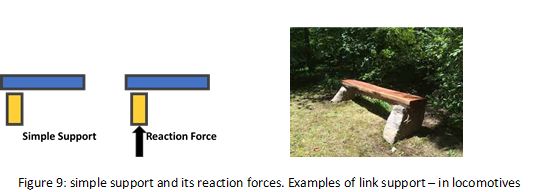1. Frictionless Support:

It is a type of support used to constrain degrees of freedom in normal directions. This support or boundary condition is used to prevent one or more flat or curved faces from moving or deforming in the normal direction as shown in fig 10. If this boundary condition is applied to the face of the body, then no portion of the surface body can move, rotate, or deform normal to the face, but for tangential directions, the surface body is free to move, rotate, and deform tangentially to the face. If this support is used for a flat surface body or solid body, then it is equivalent to the mirror symmetry condition as in fig10.